## Python Workshop: MatplotlibBased on:

This git of Zhiya Zuo

In :
import numpy as np
import matplotlib.pyplot as plt

figsize = (10, 10)


## Introduction

Visualization is one of the most important things of data analysis. Besides just producing ___readable___ plots, we should make an effort to improve the overall attractiveness of the plots. matplotlib is a powerful package for ___Python___ users. Let's start with an example.

### Anatomy of a Figure

Before we go deeper, let's take a look at the structure of a figure in matplotlib:## Line plot

First we generate sample data

In :
np.random.seed(1234)

X_arr = np.arange(10)
Y_arr = 3 * X_arr + 2 + np.random.random(size=X_arr.size)  # linear with some noise
print(X_arr)
print(Y_arr)

[0 1 2 3 4 5 6 7 8 9]
[ 2.19151945  5.62210877  8.43772774 11.78535858 14.77997581 17.27259261
20.27646426 23.80187218 26.95813935 29.87593263]


To plot a simple scatter plot, we can use plt.scatter() function

In :
plt.figure(figsize=figsize)
plt.plot(X_arr, Y_arr)
plt.title("My First Plot")
plt.show()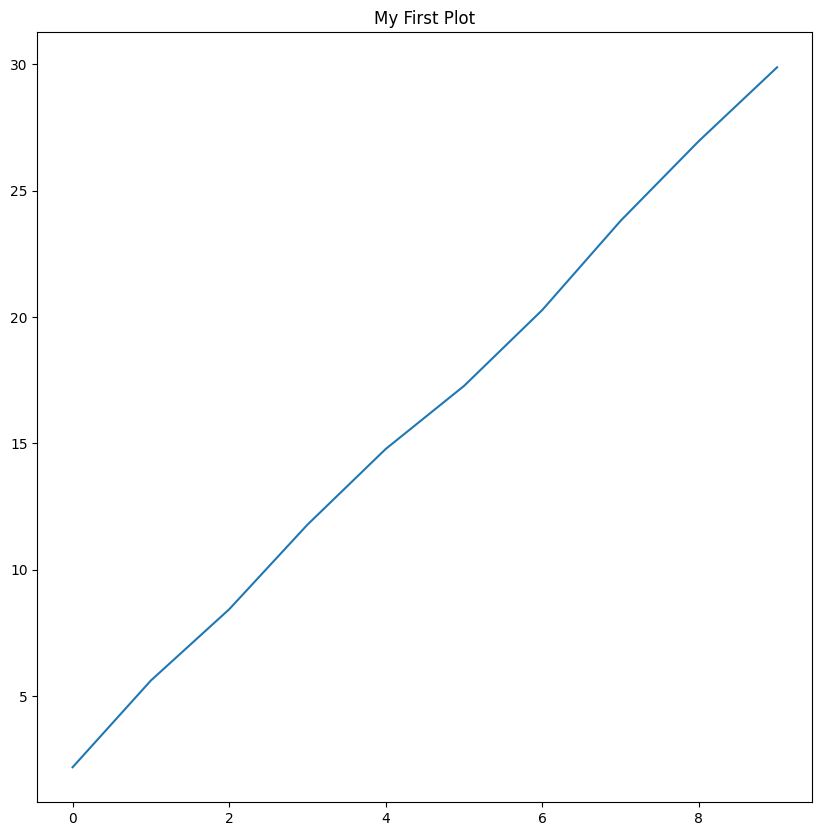## Scatter plot

In :
plt.figure(figsize=figsize)
# Use + as marker; color set as g (green); size proportion to Y values
plt.scatter(X_arr, Y_arr, marker="+", c="g")
# How about adding a line to it? Let's use plt.plot()
# set line style to dashed; color as r (red)
plt.plot(X_arr, Y_arr, "--r")
# set x/y axis limits: first two are xlow and xhigh; last two are ylow and yhigh
plt.axis([0, 10, 0, 35])
# set x/y labels
plt.xlabel("My X Axis")
plt.ylabel("My Y Axis")
# set title
plt.legend(["line", "datapoints"])
plt.title("My Second Plot")
plt.show()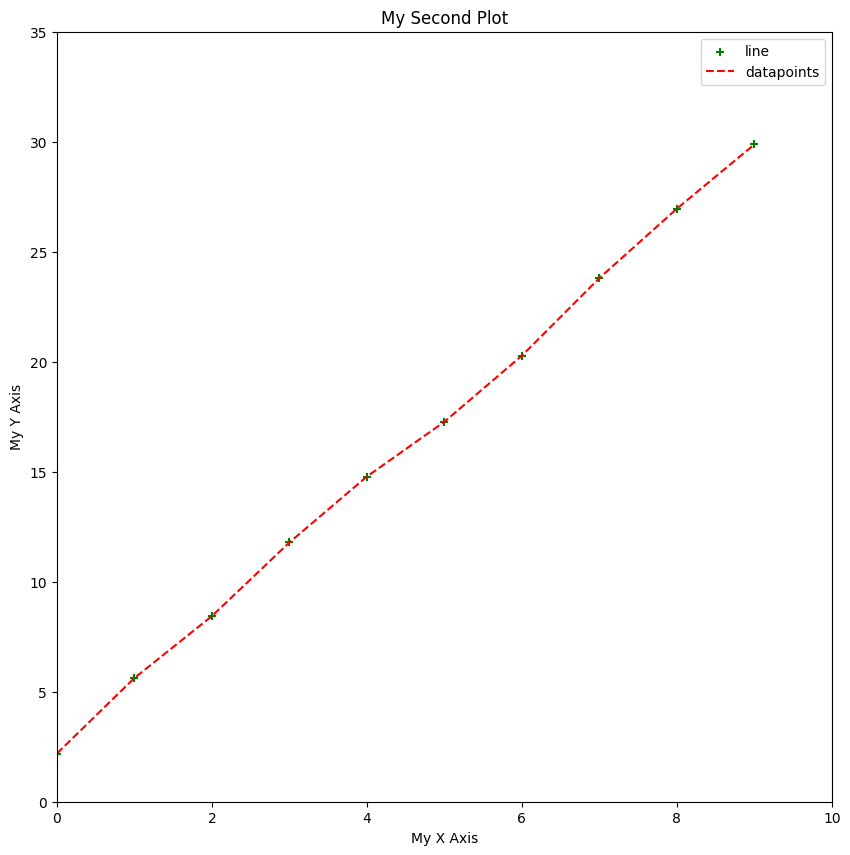## Coding style

Another possible way to work with figures in matplotlib:

In :
# plt.subplots() returns a figure object (which is the whole thing as shown above)
# and axes that control specific plots in the figure.
# Here our "subplots" layout is by default 1 row and 1 col and therefore 1 plot
fig, ax = plt.subplots(figsize=figsize)

# plot should be done on the axis: ax
ax.plot(X_arr, Y_arr)
ax.set_title("plotting with plt.subplots() is pretty much the same")
plt.show()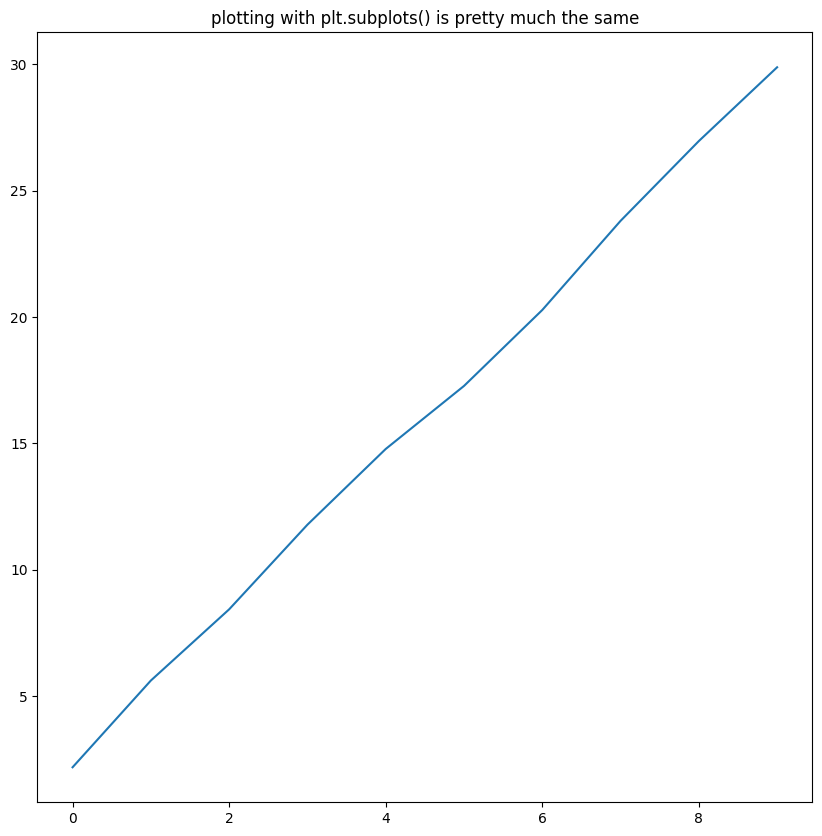Applying what we did earlier:

In :
fig, ax = plt.subplots(figsize=figsize)
# What we just did, applying to ax
ax.scatter(X_arr, Y_arr, marker="+", c="g", s=Y_arr * 10)
ax.plot(X_arr, Y_arr, linestyle="dashed", color="k")
ax.axis([0, 10, 0, 35])
ax.set_xlabel("My X Axis")
ax.set_ylabel("My Y Axis")
ax.set_title("My First Plot")
plt.show()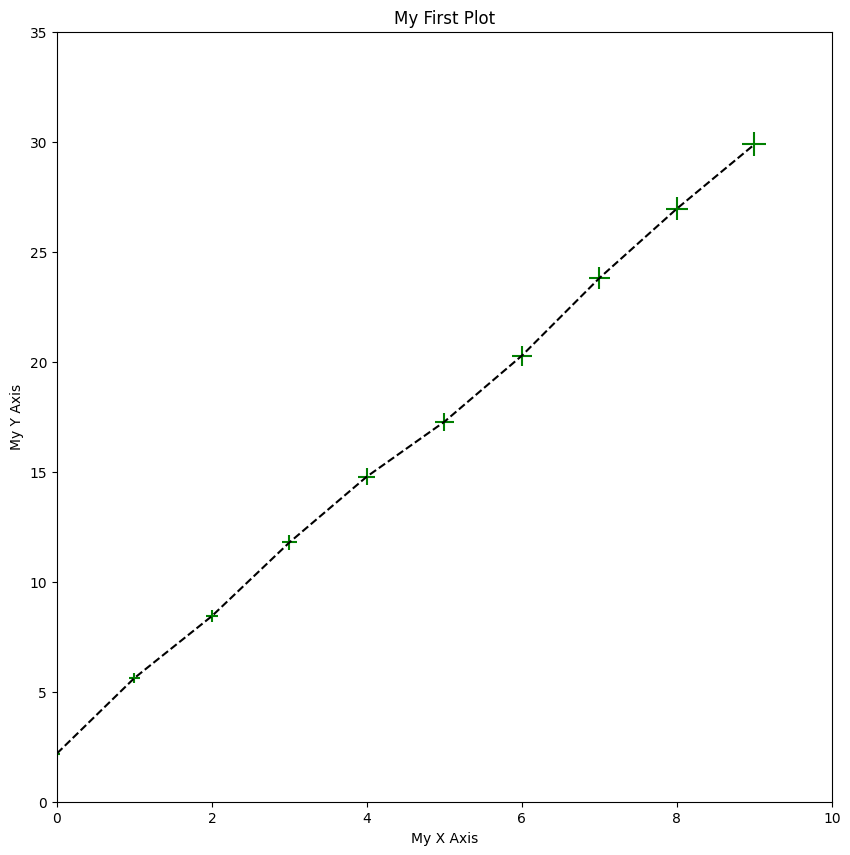This is especially useful when handling multiple plots in one figure.

In :
# Now the returned ax_arr would be np array with a shape a 2x3
fig, ax_arr = plt.subplots(2, 3, figsize=figsize)
ax_arr[0, 0].plot(X_arr, Y_arr)
ax_arr[0, 1].scatter(X_arr, Y_arr)
fig.suptitle("my subplots")
plt.show()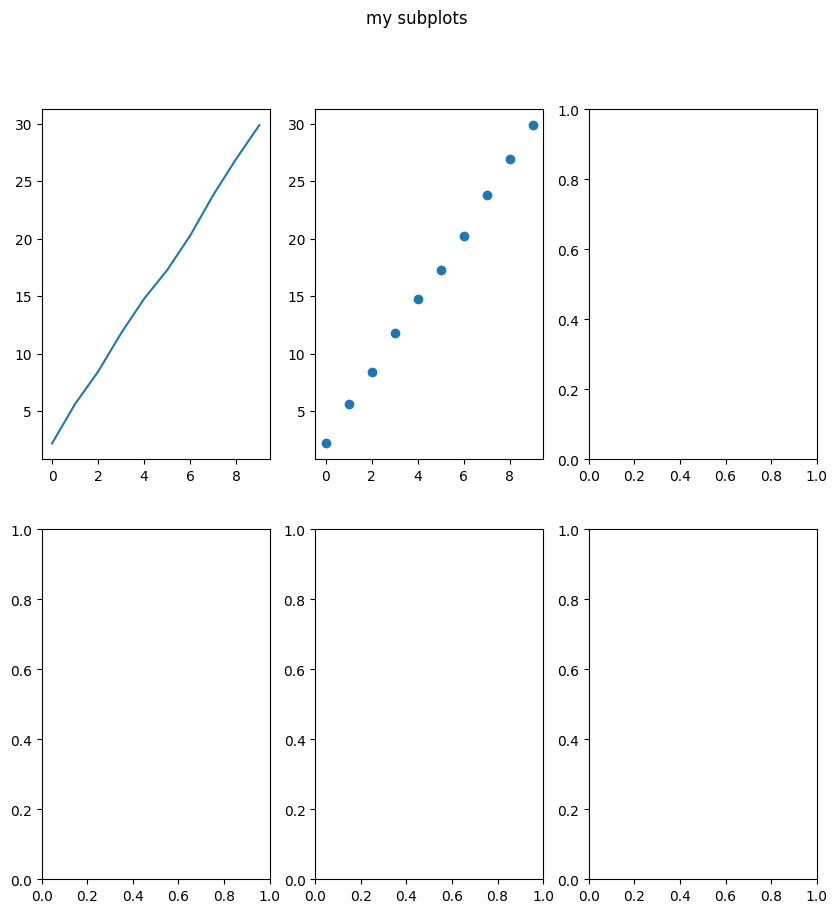## Histogram

Let's use a Gaussian distribution for illustration

In :
mu, sigma = 15, 1
gaussian_arr = np.random.normal(mu, sigma, size=10000)
np.mean(gaussian_arr), np.std(gaussian_arr, ddof=1)

Out:
(15.016359581910606, 0.9950213767631108)
In :
fig, ax = plt.subplots(figsize=figsize)
# hist() will return something but we usually do not need.
freq_arr, bin_arr, _ = ax.hist(gaussian_arr)
ax.set_title("Histogram")
plt.show()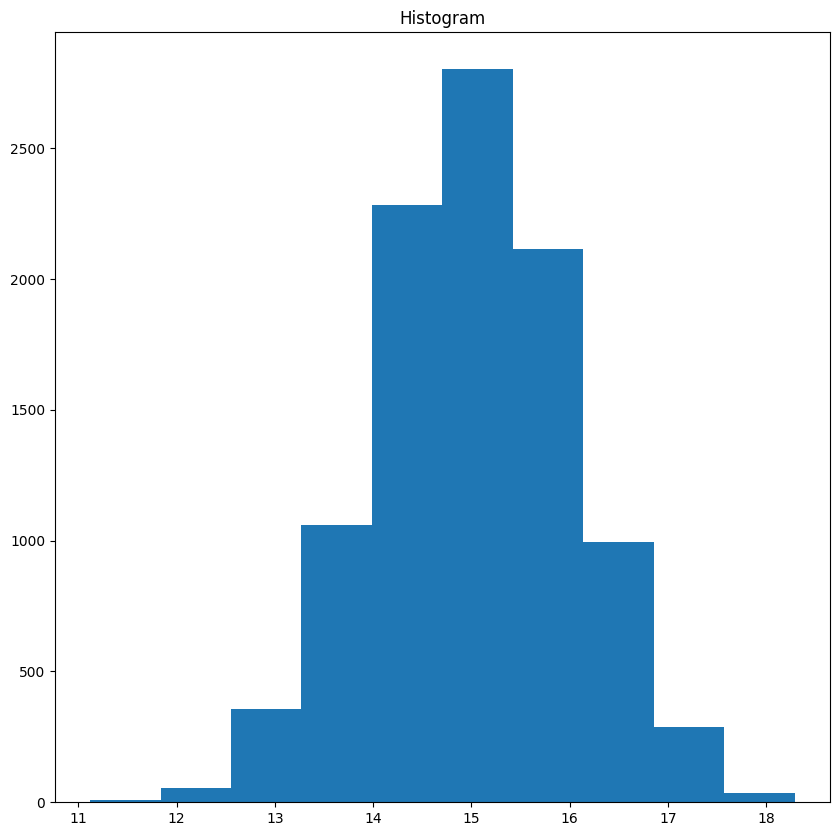We can actually customize and make it prettier

In :
fig, ax = plt.subplots(figsize=figsize)
# Facecolor set to green; transparency (alpha) level: 30%
freq_arr, bin_arr, _ = ax.hist(gaussian_arr, facecolor="g", alpha=0.3)
ax.grid()
ax.set_title("Histogram- some more features")
plt.show()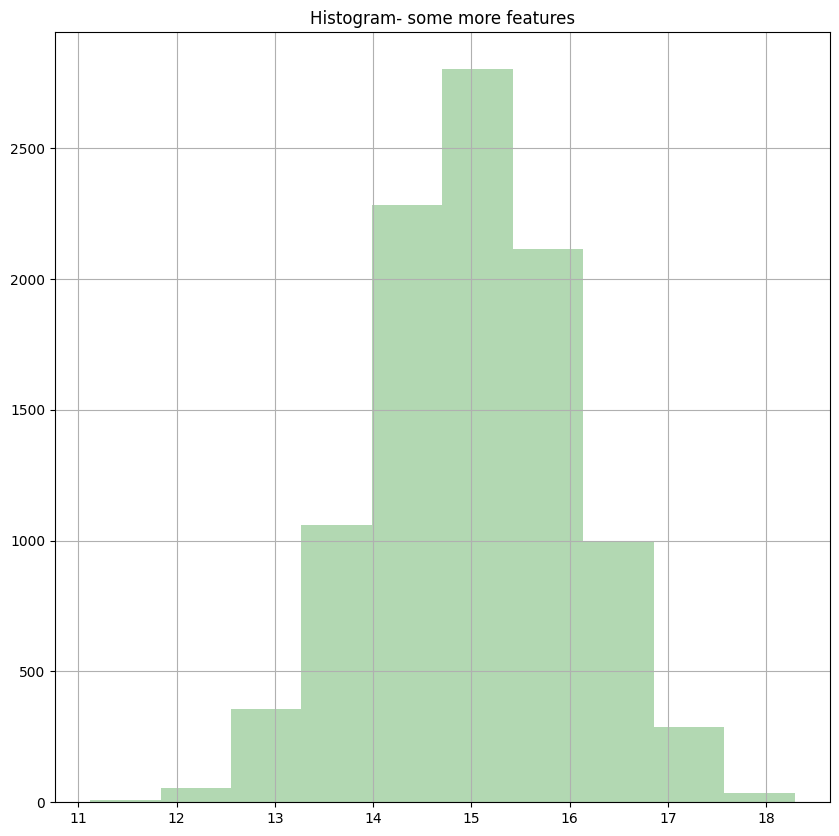## 3D plots

In :
from mpl_toolkits.mplot3d import Axes3D

fig = plt.figure(figsize=figsize)
ax = fig.gca(projection="3d")  # get current axis
theta = np.linspace(-4 * np.pi, 4 * np.pi, 100)
z = np.linspace(-2, 2, 100)
r = z ** 2 + 1
x = r * np.sin(theta)
y = r * np.cos(theta)
ax.plot(x, y, z, label="parametric curve")
ax.legend()
plt.title("3d plot")
plt.show()

C:\Users\chech\AppData\Local\Temp\ipykernel_18088\2591972693.py:5: MatplotlibDeprecationWarning: Calling gca() with keyword arguments was deprecated in Matplotlib 3.4. Starting two minor releases later, gca() will take no keyword arguments. The gca() function should only be used to get the current axes, or if no axes exist, create new axes with default keyword arguments. To create a new axes with non-default arguments, use plt.axes() or plt.subplot().
ax = fig.gca(projection="3d")  # get current axis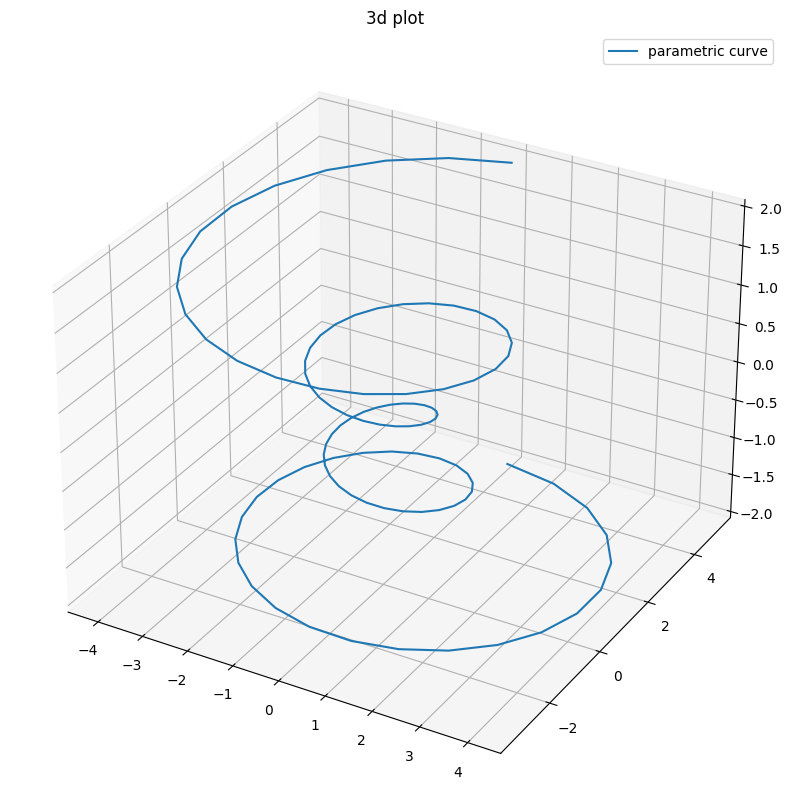## Note on IDE plotting

In regular IDE plotting, after each plot one should put

plt.show()


to show the pop-up plot window in which one can interact with the plots (zoom, rotate, etc.), and this will stop the run of your program until closed.

A way to overcome this is running:

plt.show(block=False)


but then when the script completes, all figures are closed...

My preferred solution is running with block=False to all figures except the last one.